Project tutorial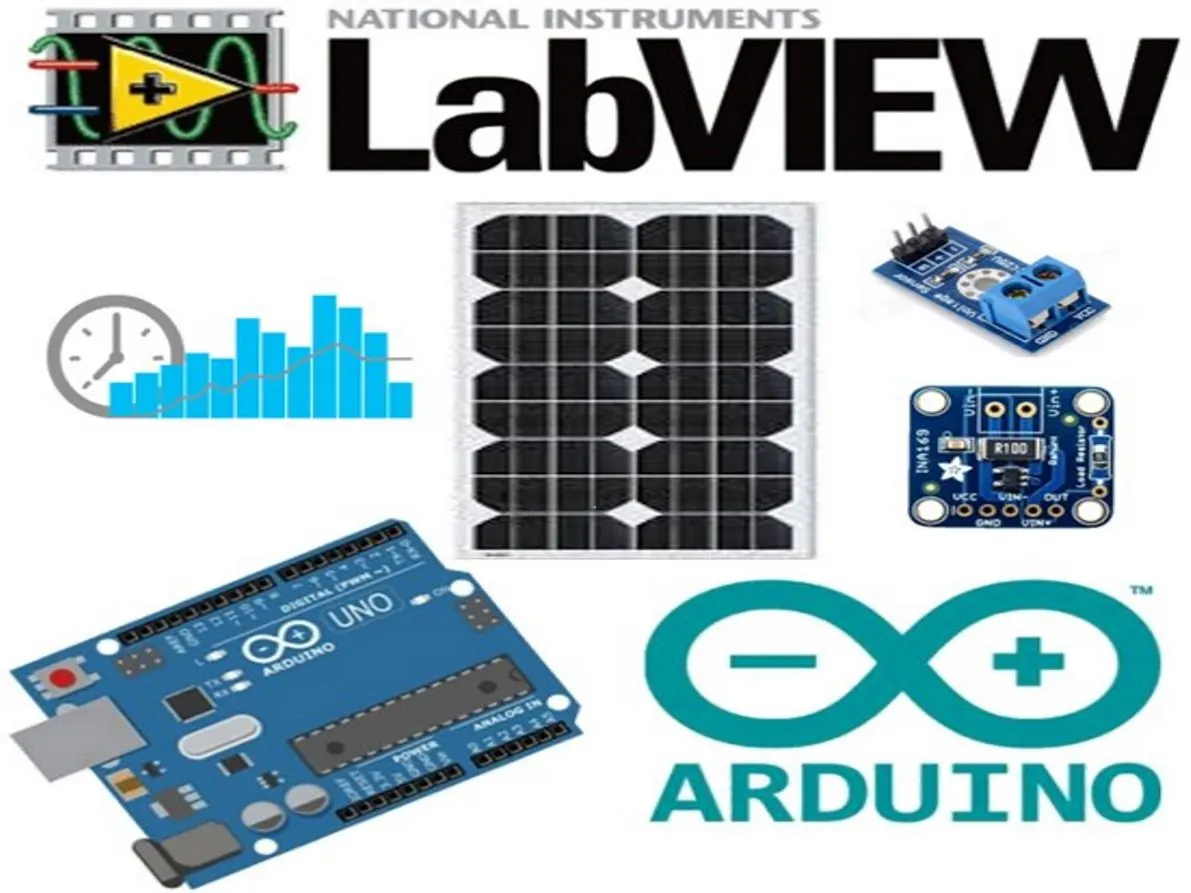# Solar Panel Data Monitoring using Arduino and LabView © MIT

This project presents a simple virtual instrument system based on LabVIEW and Arduino to characterize and monitor a PV panel.

• 11,093 views
• 1 comment
• 12 respects

## Components and suppliesArduino UNO
×1
 Small Mono TDC-M20-25-36
×1
 Adafruit B25 0 to 25V Voltage Sensor Module
×1
 Adafruit INA169 Analog DC Current Sensor
×1

## Necessary tools and machines

 Arduino IDE
 nanoFramework NI LabVIEW 2015

## About this project

This project proposes a low-cost real-time virtual instrumentation system based on LabVIEW and Arduino to characterize a PV panel. Also for monitoring its output data (current, voltage, and power) under real condition. A PV panel, Arduino UNO board, voltage, and current sensors are used as hardware components. Arduino IDE, LabVIEW, and LIFA are used as the software of the system design.

The Figure below shows the schematic diagram of the instrumentation system. The current and voltage sensors sense the output current and voltage from the PV panel. Then, the readings of the two sensors are transmitted to the microcontroller of the Arduino UNO board. While the LabVIEW Interface for Arduino (LIFA) serves as an interface between the Arduino microcontroller and computer to make communication between the microcontroller and LabVIEW through a serial connection. During the acquisition process, the measured data of the current, voltage and power are plotted directly in a monitoring platform developed under LabVIEW.

This project is linked to this research paper.

Explanatory video:

## Code

##### Real-time data acquisition of solar panel using Arduino and LabVIEWScheme
Block diagram and the interface of the developed system under LabVIEW
`No preview (download only).`

## Schematics

For measuring the PV panel's output voltage, the B25 voltage sensor module is used (Figure (a)). The B25 voltage sensor uses the principle of the voltage divider, it is essentially a voltage divider using a resistance of 30 kΩ and a resistance of 7.5 kΩ as shown in Figure (b). It is used to reduce the input voltage up to 5 times compared to the original voltage because the maximum analog input voltage of the Arduino microcontroller is 5 V. The voltage sensor module will be installed in parallel with the PV panel load as shown in Figure (c). The sensor reading is a digital value ( Vout) which varies between 0 and 1023. Since the ADC of the microcontroller is coded in 10 bits, the resolution of the PV panel module voltage is 0.00489 V (5/1023) and the input voltage of this module must be more than 0.02445 V (0.00489 V× 5). Hence, the output voltage of the PV panel can be formulated as the following equation: V=5*Vout*(5/1023)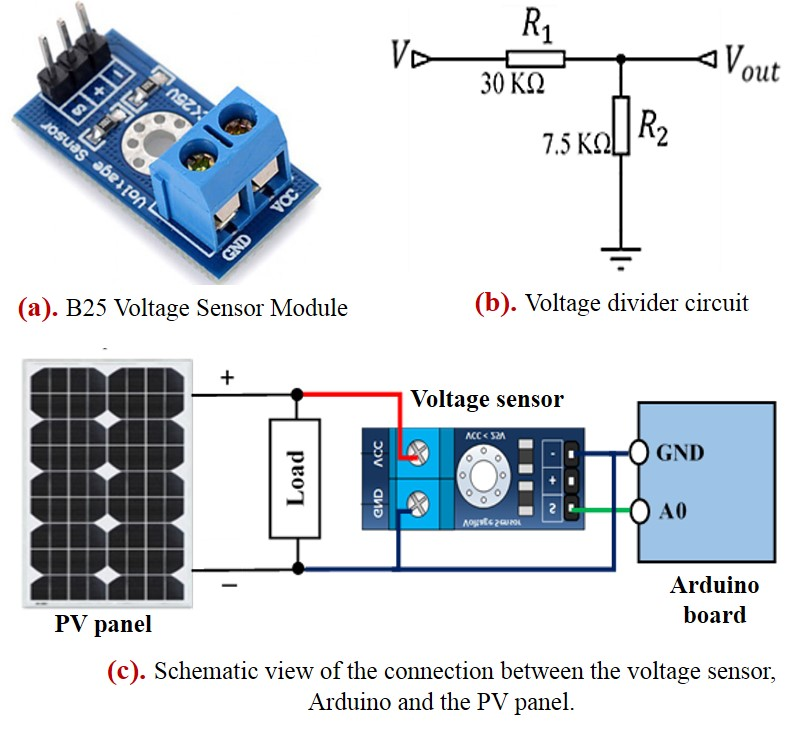The current sensor used to sense the PV panel output current is the INA169 module (Figure (a)), it can measure a continuous current up to 5 A. Figure (b) shows the INA169 current sensor circuit (from INA169 Datasheet). The INA169 is a high-side current monitor that measures the voltage drop across a sense resistor (Rs). Then, a voltage level (Vo) is generated at the output resistor (RL). Therefore, as shown in Figure (b), the output current of the sensor module can be obtained by the following equation:
Is = (Vo*1K) / (Rs*Rl)
Where:
- Vo: is the voltage we measured at the output of the INA169.
- 1kΩ is a constant resistance value we need to include due to the
internals of the INA169.
- Rs: is the value of the shunt resistor, this is set at 0.1 Ω.
- RL: is the value of the output resistor, this is set at 10 KΩ.
Hence, the output current of the PV panel can be formulated as the following equation:
I =( Vo*5) / 1023
The output voltage of the INA169 module (Vo) is the input voltage at an Arduino analog pin (A1), which varies between 0 and 1023. The current sensor module must be connected in series to the positive side of the PV panel and that of the load as shown in Figure (c).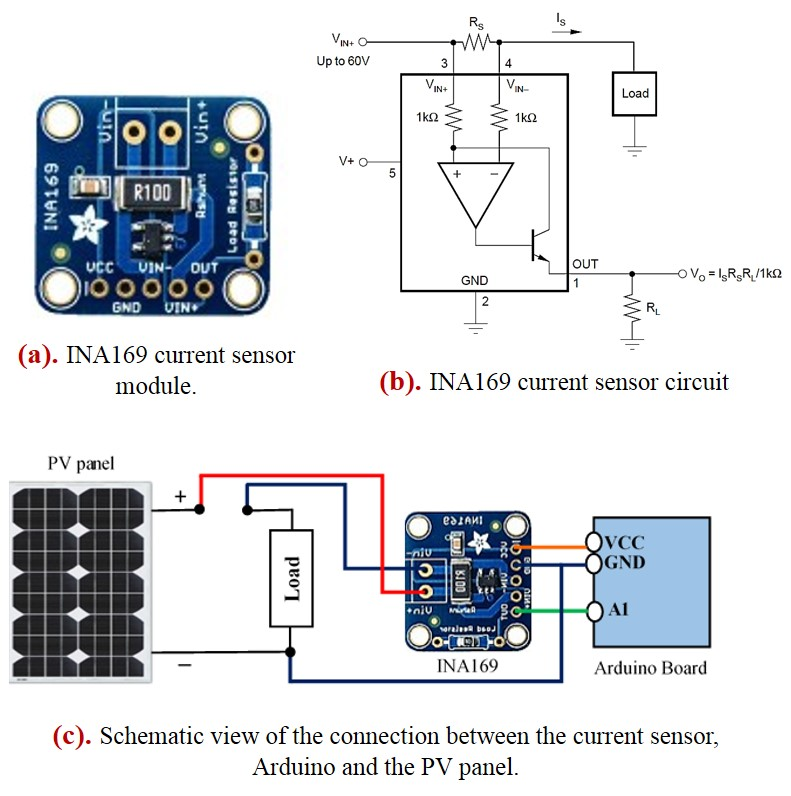To send data from Arduino to LabVIEW, the LIFA is necessary to make the connection between the Arduino microcontroller and the computer. For this, you just need to follow the following steps:
+ Install the LabVIEW software (LabVIEW 2011 or later);
+ Download the NI-VISA drivers and install it. Since the Arduino appears as a serial instrument device for LabVIEW, the NI-VISA drivers must be downloaded and installed to communicate with Arduino board in LabVIEW;
+ Download the JKI VI Package Manager (VIPM) and install it, it allows to install and update LabVIEW libraries;
+ Install the LIFA by following the next steps:
Lunch LabVIEW → click on tool → go to VIPM → browse to LIFA in the list of packages → click on the Install Upgrade Packages button;
+ Download the open source Arduino IDE software and install it;
+ The LIFA provides a sketch program that must be uploaded to the Arduino before you can use the virtual instruments to communicate with it. For this, Open the Arduino IDE application and following the next steps to uploading LIFA firmware to the Arduino board:
=> Go to File → Click on Open → go to the sketch which is located at: C:\ProgramFiles(x86)\National Instruments\LabVIEW 2015\vi.lib\LabVIEW Interface for Arduino\Firmware\LIFA_Bas;
=> Select the type of used Board (Arduino UNO);
=> Connect the board to computer via USB cable and select the used serial port;
=> Verify and Upload the sketch program LIFA to the Arduino microcontroller;
+ After that, you can start building your code in Arduino with LIFA Block Diagram.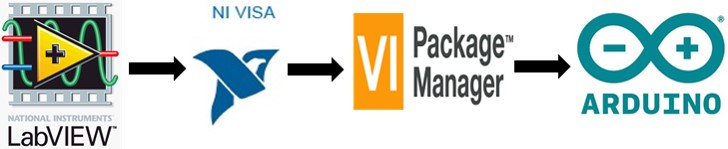The overview of the block diagram and the front panel of the virtual instrumentation system is shown in the Figure below. For enriching understanding, the block diagram is separated into four parts. The first one illustrates the blocks of initialization and configuration of the serial connection between the hardware (Arduino UNO) and software (LabVIEW), that by defining the type of used Arduino (Arduino UNO), type of serial communication (USB) and the Baud Rate (9600 bits per second). In the second part, the voltage and current readings will be obtained from the microcontroller by using the analog read function in LIFA's palette in which the initialization of the selected Arduino pins as inputs was made, A0 pin for voltage reading and A1 pin for current reading. The third part shows that the current, voltage and output power readings are carried out and will be plotted directly according to time by using scrolling graphs in the LabVIEW palette. Note that the reading of the voltage is multiplied by 5 because as it is demonstrated in the hardware part that the voltage reading by the microcontroller varies between 0 and 5 V, it is necessary to multiply it by 5 to obtain the PV panel's output voltage. The PV panel's output power reading is done out by the product of the current and voltage reading. Finally, in the last part, the I-V and P-V curves of PV panel characteristics will be plotted in the monitoring interface of the system by using x/y graphs in the LabVIEW palette.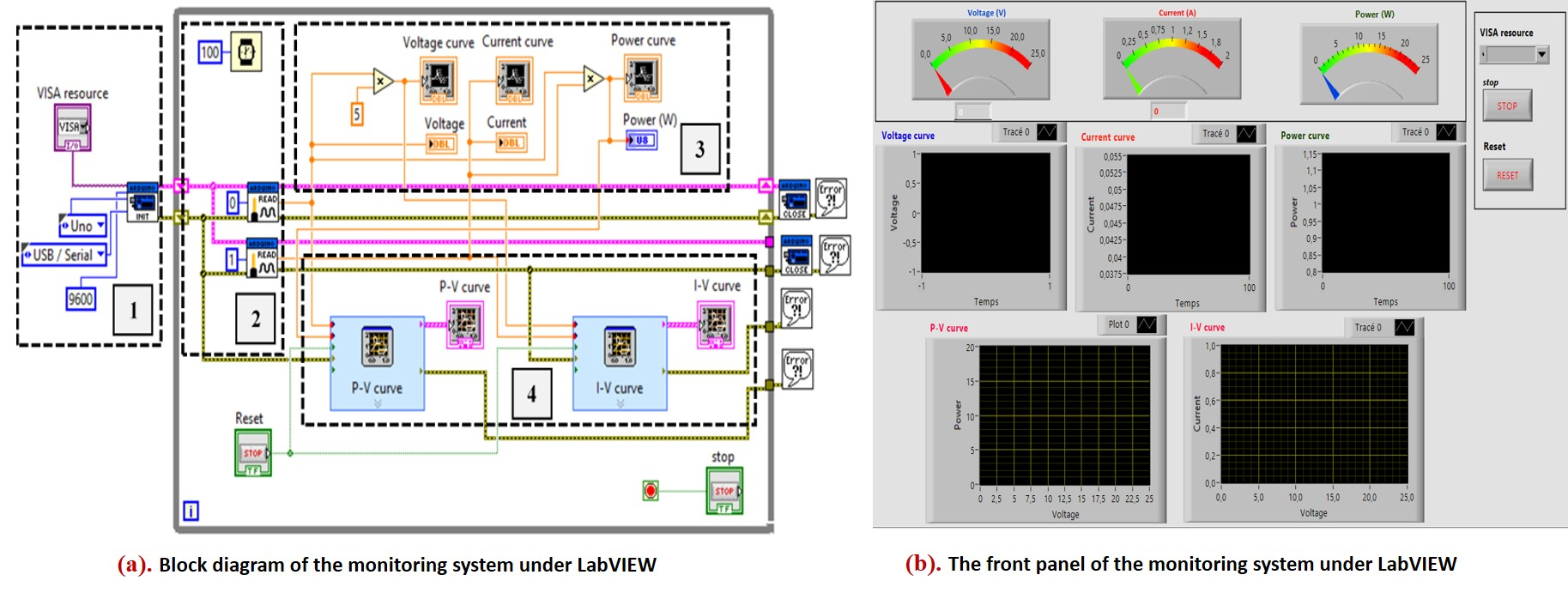In the Figure below, the experimental setup of the real-time virtual instrumentation system is shown. Apart PV panel, Arduino UNO board, voltage and current sensor, different components are used in the experimental setup such us lamps of 100 W that act as a solar simulator, a variable resistance between 0 and 300 Ω as a load and acting as a light intensity driving. The microcontroller's operation is programmed in the block diagram to measure the values relative to the current and the voltage captured by the sensors successively every 100 ms. Once the Arduino board is connected to the computer through USB cable, we launch the software interface of our system under LabVIEW. Then, we defined in the visa block the serial port where the Arduino board is connected to the computer. Thereafter, after the execution of the program, the output data will be plotted in real-time on the monitoring interface under LabVIEW.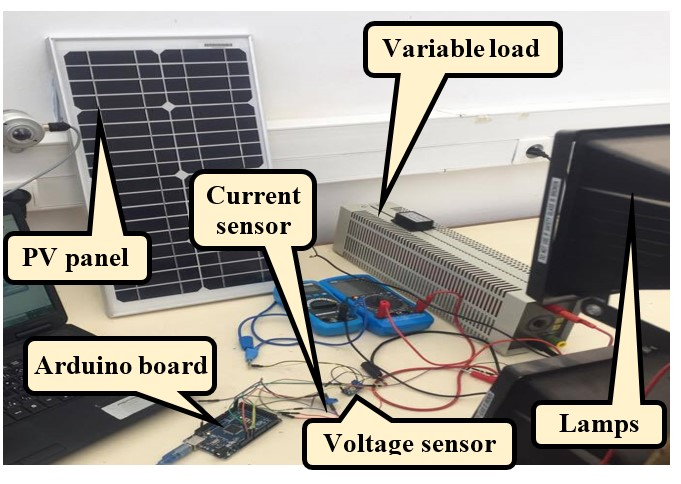The results of the direct monitoring of PV panel output data and the I-V/ P-V curves of the PV panel are shown in the Figure below.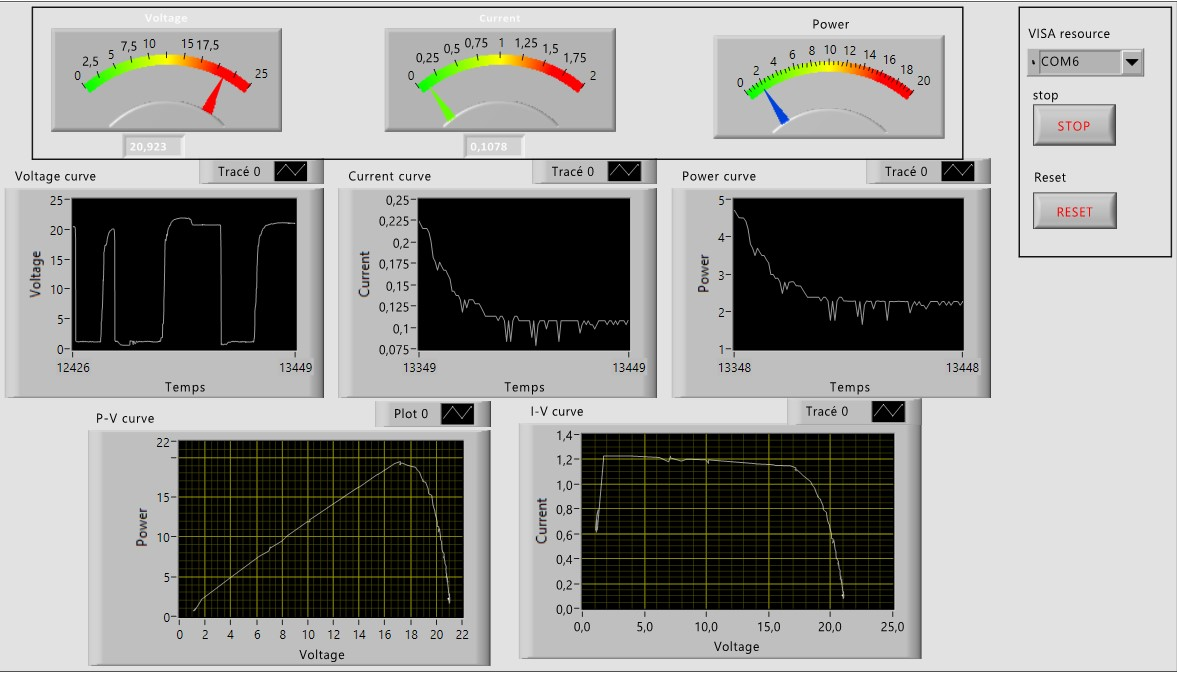## Comments

#### Team members##### Aboubakr_El Hammoumi
• 4 projects
• 20 followers• 4 projects
• 22 followers

March 28, 2020

#### Members who respect this project

See similar projects
you might like

#### Real-Time Data Acquisition of Solar Panel Using Arduino

Project tutorial by Saad Motahhir and Aboubakr_El Hammoumi

• 13,779 views
• 3 comments
• 22 respects

#### Helium Solar Monitoring Board with Relays

Project tutorial by Jade Perreault

• 4,731 views
• 2 comments
• 18 respects

#### Dual Axis Solar Tracker Panel with Auto and Manual Mode

Project tutorial by Giannis Arvanitakis

• 40,749 views
• 13 comments
• 87 respects

#### Servo-Controlled, Light-Tracking Solar Panel Platform

Project tutorial by scott mangiacotti

• 23,128 views
• 12 comments
• 60 respects

#### Solar Panel Sun Tracker - Phone Charger

Project tutorial by FIELDING

• 87,678 views
• 24 comments
• 251 respects

#### Solar Tracker with Live Data Feed - Windows IoT

Project tutorial by Jed Hodson

• 20,648 views
• 1 comment
• 33 respects